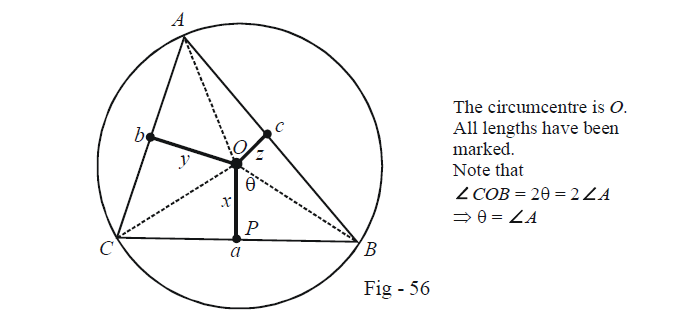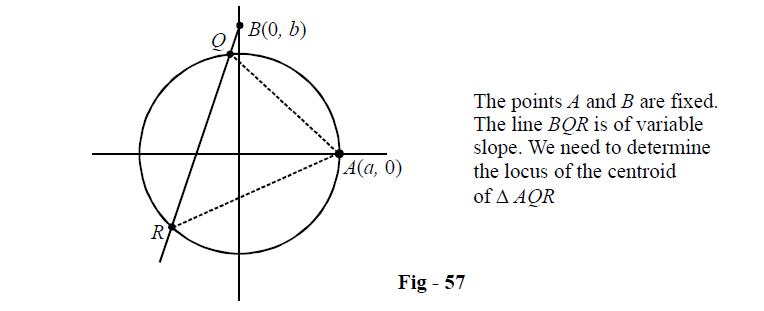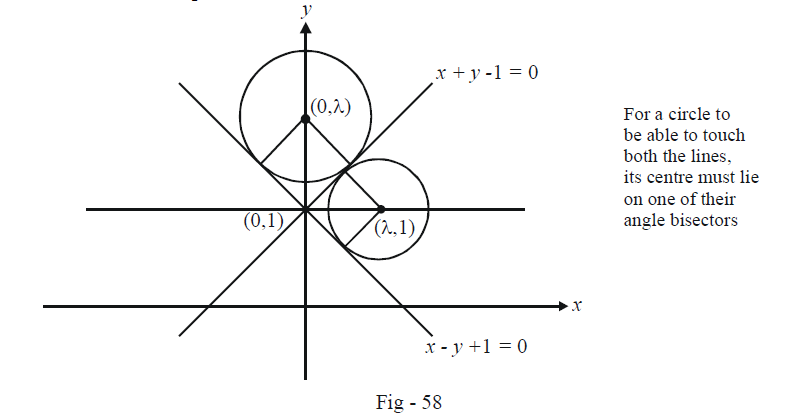# Circles Set-4

Go back to  'SOLVED EXAMPLES'

Example –10

Let x, y and z be the lengths of the perpendiculars dropped from the circumcentre in a triangle ABC to the sides BC, CA, and AB respectively. The lengths of BC, CA and AB are a, b and c respectively. Show that

$\frac{a}{x} + \frac{b}{y} + \frac{c}{z} = \frac{{abc}}{{4xyz}}$

Solution: We can attempt this question through a co-ordinate approach but the nature of the problem posed suggests that a plane geometric approach would be better since no equations need to be determined here.

Refer to the following diagram  which shows the situation clearly :In $$\Delta OPB,$$ we have

$\tan {\rm{\theta }} = \tan \angle A = \frac{a}{{2x}}\,\,\,\,\,\,\,\,\,\,\,\,\,\,\,\,\,\,\,\,\,\,\,\,\,...\left( 1 \right)$

Similarly,

\begin{align}\tan \angle B=\frac{b}{2y}\,\,\text{and}\,\,\tan \angle C=\frac{C}{2z}\text{ }\!\!~\!\!\text{ }\!\!~\!\!\text{ }\!\!~\!\!\text{ }\qquad\qquad\qquad...(2)\end{align}

Now, the angles $$\angle A,\,\,\angle B$$  and $$\angle C$$  are those of a triangle so that

\begin{align}&\qquad\quad\angle A + \angle B + \angle C = \pi \\&\Rightarrow \quad \tan (\angle A + \angle B) = \tan (\pi - \angle C)\\&\qquad\qquad\qquad\qquad\quad = - \tan \angle C\\&\Rightarrow \quad \frac{{\tan \angle A + \tan \angle B}}{{1 - \tan \angle A\tan \angle B}} = - \tan \angle C\\&\Rightarrow \quad \tan \angle A + \tan \angle B + \tan \angle C = \tan \angle A\tan \angle B\tan \angle C\end{align}

Using (1) and (2) in the relation above, we obtain the required relation.

Example –11

Let $$A(a,0)$$ be a point on the circle $${x^2} + {y^2} = {a^2}.$$  Through another point $$B(0,b),$$ chords are drawn to meet the circle at points Q and R. Find the locus of the centroid of $$\Delta AQR.$$

Solution: The following diagram shows an example of the situation described :The equation of $$BQR$$ can be written as $$y = mx + b$$ where m is variable. The intersection points of $$BQR$$ with the circle (Q and R) are given by simultaneously solving the system of equations

\begin{align}&{x^2} + {y^2} = {a^2}\\&y = mx + b\end{align}

Thus,

\begin{align}&\,\,\,\,\,\,\,\,\,\,\,\,\,\,\,\,\,{x^2} + {(mx + b)^2} = {a^2}\\&\Rightarrow \quad (1 + {m^2}){x^2} + 2mbx + {b^2} - {a^2} = 0\,\,\,\,\,\,\,\,\,\,\,\,\,\,\,...(1)\end{align}

If we assume the co-ordinates of Q and R to $$({x_1},{y_1})$$ and $$({x_2}{y_2}),$$ we have from (1)

${x_1} + {x_2} = - \frac{{2mb}}{{1 + {m^2}}}$

Thus,

${y_1} + {y_2} = m({x_1} + {x_2}) + 2b = - \frac{{2{m^2}b}}{{1 + {m^2}}} + 2b = \frac{{2b}}{{1 + {m^2}}}$

Let the centroid of $$\Delta AQR$$ be $$(h,k).$$ We have

\begin{align}&\,\,\,\,\,\,\,\,\,\,\,\,\,\,\,\,\frac{{a + {x_1} + {x_2}}}{3} = h,\,\, & \frac{{0 + {y_1} + {y_2}}}{3} = k\\&\Rightarrow\quad {x_1} + {x_2} = 3h - a,\,\, & {y_1} + {y_2} = 3k\\&\Rightarrow \quad - \frac{{2mb}}{{1 + {m^2}}} = 3h - a,\,\,\, & \frac{{2b}}{{1 + {m^2}}} = 3k\end{align}

These two relations can be used to eliminate m and obtain a relation in $$(h,k).$$  To specify the locus conventionally, we use $$(x,y)$$ instead of $$(h,k).$$ The equation obtained is (verify):

${x^2} + {y^2} - \frac{2}{3}ax - \frac{2}{3}by + \frac{{{a^2}}}{9} = 0$

Example –12

Find the equation of the family of circles touching the lines given by

${x^2} - {y^2} + 2y - 1 = 0$

Solution: The equation to the pair of lines can be factorised to yield the individual lines as

$x-y+1=0\,\,\,and\,\,\,x+y-1=0.$

From the following diagram, observe carefully that for a circle to touch both the lines above, its centre must be on one of their angle bisectors because only then will the distance of the centre from the two lines be equal.The angle bisectors can be seen from inspection to be $$x = 0$$ and $$y = 1.$$ Thus, the centre of the variable circle can be assumed to be:

 \begin{align}& (i)\qquad(0,\lambda )\text{ }\!\!~\!\!\text{ } \\ & \qquad \quad\,\,\lambda \text{ }\!\!~\!\!\text{ }\in \mathbb{R} \quad : \\ \end{align} \begin{align}& \text{The distance of}\;(0,\lambda )\;\text{from the two lines is }\frac{\left| \lambda \text{ }\!\!~\!\!\text{ }-1 \right|}{\sqrt{2}} \\ & \text{Thus, the equation of the variable circle in this case is} \\ & {{x}^{2}}+{{(y-\lambda )}^{2}}=\frac{{{(\lambda -1)}^{2}}}{2}\,\,\,\,\,\,\,\,\,\,\,\,\,\,\,\,\,\,\,\,\,\,\,\,\,\,\,\,\,\,\,\,\,\,\,\,\,\,\,\,\,\,\,\,\,\,\,.....(1) \\ \end{align} \begin{align}& (ii)\qquad(\lambda,1 )\text{ }\!\!~\!\!\text{ } \\ & \qquad \quad\,\,\lambda \text{ }\!\!~\!\!\text{ }\in \mathbb{R} \quad :\\ \end{align} \begin{align}& \text{ }\!\!~\!\!\text{ The distance of }\,(\lambda ,1)\,\text{from the two lines in }\frac{|\lambda |}{\sqrt{2}} \\ & \text{Thus, the equation of the variable circle in this case is} \\ & {{(x-\lambda )}^{2}}+{{(y-1)}^{2}}=\frac{{{\lambda }^{2}}}{2}\,\,\,\,\,\,\,\,\,\,\,\,\,\,\,\,\,\,\,\,\,\,\,\,\,\,\,\,\,\,\,\,\,\,\,\,\,\,\,\,\,\,.....(2) \\ \end{align}

The equations (1) and (2) represent the required family of circles.

Learn from the best math teachers and top your exams

• Live one on one classroom and doubt clearing
• Practice worksheets in and after class for conceptual clarity
• Personalized curriculum to keep up with school